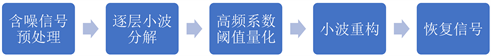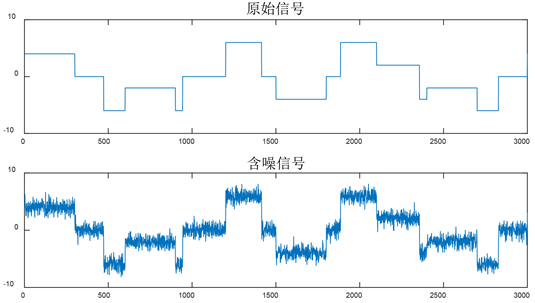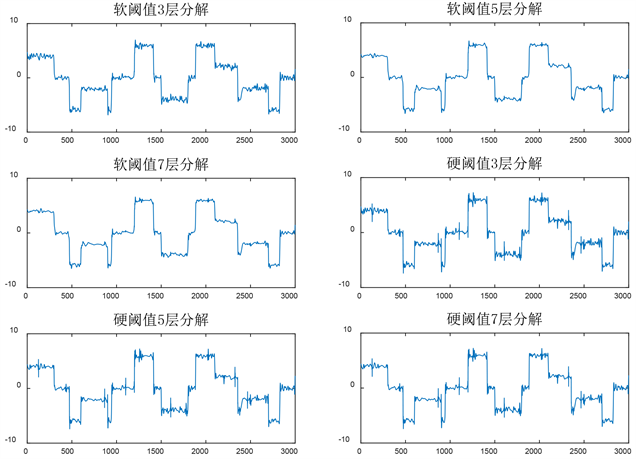# 基于小波分析的信号去噪研究Research on Signal Denoising Based on Wavelet Analysis

DOI: 10.12677/AAM.2021.104142, PDF, HTML, XML, 下载: 20  浏览: 84  科研立项经费支持

Abstract: This paper, through the theory of wavelet analysis of non-stationary signal containing noise denoising research, analyzes the common principle and characteristics of signal filtering denoising method, discusses the denoising method based on wavelet analysis, uses MATLAB software for different denoising methods to carry out simulation experiments, and finally makes a conclusion of the several methods of the above.

1. 引言

2. 非平稳信号

$m{x}_{1}\left(t\right)+n{x}_{2}\left(t\right)\to m{y}_{1}\left(t\right)+n{y}_{2}\left( t \right)$

3. 一些常见的信号去噪方法及其特点

3.1. 均值滤波

$g\left(x,y\right)=\frac{1}{S}\underset{\left(x,y\right)\in U}{\sum }f\left(x,y\right)$ (1)

3.2. 中值滤波

3.3. 快速傅里叶变换

4. 小波分析

1) 先要对含噪信号进行小波分解，我们先选择一个合适的小波基函数和要对它进行分解的层次k，这里k是一个正整数，然后计算出含噪信号到第k层的分解。

2) 然后是对信号的高频系数进行阈值量化处理，在每一层中选择一个合适阈值对该层的高频系数进行量化。

3) 最后是对含噪信号的小波重构，我们根据小波分解过后的第k层的低频系数和经过处理后的从第1层到第k层的高频系数来计算它的小波重构。Figure 1. Flow chart of wavelet denoising

5. 仿真实验与结果对比

5.1. 信号去噪方法的对比Figure 2. Original signal and noisy signalFigure 3. Comparison of various denoising methodsTable 1. Signal quality evaluation index of different denoising methods

5.2. 不同参数对小波方法去噪的影响Table 2. Signal evaluation index of different parameters in wavelet methodFigure 4. Influence of various parameters on wavelet denoising

6. 结束语

  (美) Sanjit K. Mitra. 数字信号处理——基于计算机的方法[M]. 第4版. 余翔宇, 译. 北京: 电子工业出版社, 2018.  史洁玉. MATLAB信号处理超级学习手册[M]. 北京: 人民邮电出版社, 2014: 212.  (美) Alan V. Oppenheim, Alan S. Willsky, S. Hamid Nawab. 信号与系统[M]. 第2版. 刘树棠, 译. 北京: 电子工业出版社, 2020.  (美) Rafael C. Gonzalez, Richard E. Woods. 数字图像处理[M]. 第4版. 阮秋琦, 阮宇智, 译. 北京: 电子工业出版社, 2020.  (美) Albert Boggess, Francis J. Narcowich. 小波与傅里叶分析基础[M]. 第2版. 芮国胜, 康健, 译. 北京: 电子工业出版社, 2017.  刘志松. 基于小波分析的信号去噪方法[J]. 浙江海洋学院学报(自然科学版), 2011, 30(2): 150-154.  曹银萍, 郭璐. 基于MATLAB的小波分析在信号去噪中的应用[J]. 信息记录材料, 2018, 19(7): 85-87.  申莎莎. 基于小波变换与傅里叶变换对比分析及其在信号去噪中的应用[J]. 山西师范大学学报(自然科学版), 2018, 32(3): 27-32.  Mallat, S. and Hwang, W.L. (1992) Singularity Detection and Processing with Wavelets. IEEE Transactions on Information Theory, 38, 617-643.  韦力强. 基于小波变换的信号去噪研究[D]: [硕士学位论文]. 长沙: 湖南大学, 2007.